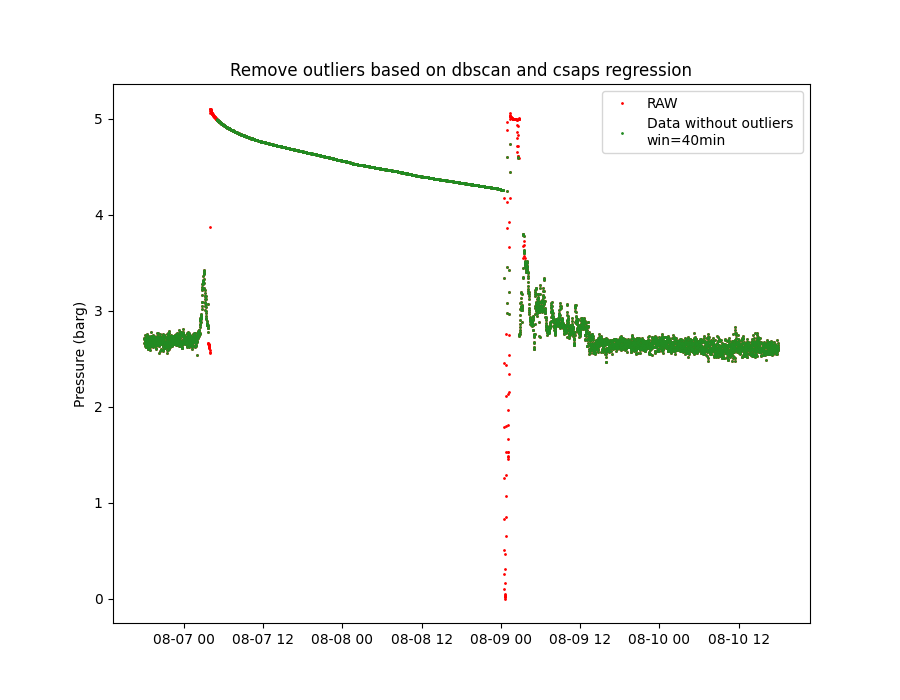# Outlier detection with DBSCAN and spline regression

Example of outlier detection from time series data using DBSCAN and spline regression. We use data from a compressor suction pressure sensor. The data is in barg units and resampled to 1 minute granularity. The figure shows the data without outliers considering a time window of 40min.```import os

import matplotlib.pyplot as plt
import pandas as pd

from indsl.statistics import remove_outliers

# TODO: USe a better data set to show how the outlier removal. Suggestion, use a synthetic data set.

base_path = "" if __name__ == "__main__" else os.path.dirname(__file__)
data = data.squeeze()
data.index = pd.to_datetime(data.index)

plt.figure(1, figsize=[9, 7])
plt.plot(data, ".", markersize=2, color="red", label="RAW")

# Remove the outliers with a time window of 40min and plot the results
plt.plot(
remove_outliers(data, time_window="40min"),
".",
markersize=2,
color="forestgreen",
label="Data without outliers \nwin=40min",
)

plt.ylabel("Pressure (barg)")
plt.title("Remove outliers based on dbscan and csaps regression")
_ = plt.legend(loc=1)
plt.show()
```

Total running time of the script: ( 0 minutes 0.232 seconds)

Gallery generated by Sphinx-Gallery